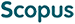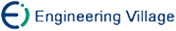梁吉业, 白 亮, 曹付元. 基于新的距离度量的K-Modes聚类算法[J]. 计算机研究与发展, 2010, 47(10): 1749-1755.
 引用本文: 梁吉业, 白 亮, 曹付元. 基于新的距离度量的K-Modes聚类算法[J]. 计算机研究与发展, 2010, 47(10): 1749-1755.Liang Jiye, Bai Liang, Cao Fuyuan. K-Modes Clustering Algorithm Based on a New Distance Measure[J]. Journal of Computer Research and Development, 2010, 47(10): 1749-1755.
 Citation: Liang Jiye, Bai Liang, Cao Fuyuan. K-Modes Clustering Algorithm Based on a New Distance Measure[J]. Journal of Computer Research and Development, 2010, 47(10): 1749-1755.## K-Modes Clustering Algorithm Based on a New Distance Measure

• 摘要: 传统的K-Modes聚类算法采用简单的0-1匹配差异方法来计算同一分类属性下两个属性值之间的距离, 没有充分考虑其相似性. 对此, 基于粗糙集理论, 提出了一种新的距离度量. 该距离度量在度量同一分类属性下两个属性值之间的差异时, 克服了简单0-1匹配差异法的不足, 既考虑了它们本身的异同, 又考虑了其他相关分类属性对它们的区分性. 并将提出的距离度量应用于传统K-Modes聚类算法中. 通过与基于其他距离度量的K-Modes聚类算法进行实验比较, 结果表明新的距离度量是更加有效的.

Abstract: The leading partitional clustering technique, K-Modes, is one of the most computationally efficient clustering methods for categorical data. In the traditional K-Modes algorithm, the simple matching dissimilarity measure is used to compute the distance between two values of the same categorical attributes. This compares two categorical values directly and results in either a difference of zero when the two values are identical or one if otherwise. However, the similarity between categorical values is not considered. In this paper, a new distance measure based on rough set theory is proposed, which overcomes the shortage of the simple matching dissimilarity measure and is used along with the traditional K-Modes clustering algorithm. While computing the distance between two values of the same categorical attributes, the new distance measure takes into account not only their difference but also discernibility of other relational categorical attributes to them. The time complexity of the modified K-Modes clustering algorithm is linear with respect to the number of data objects which can be applied for large data sets. The performance of the K-Modes algorithm with the new distance measure is tested on real world data sets. Comparisons with the K-Modes algorithm based on many different distance measures illustrate the effectiveness of the new distance measure./下载:  全尺寸图片 幻灯片
• 分享
• 用微信扫码二维码

分享至好友和朋友圈